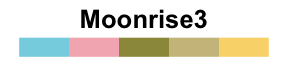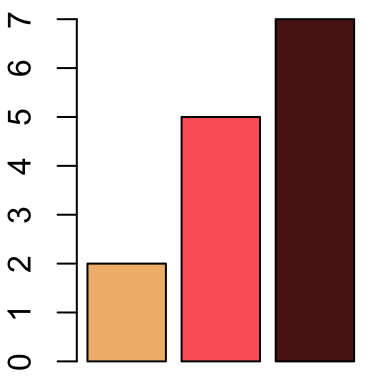# Colors in R

In R, colors can be specified either by name (e.g col = “red”) or as a hexadecimal RGB triplet (such as col = “#FFCC00”). You can also use other color systems such as ones taken from the RColorBrewer package.

# Built-in color names in R

We will use the following custom R function to generate a plot of color names available in R :

``````# Generate a plot of color names which R knows about.
#++++++++++++++++++++++++++++++++++++++++++++
# cl : a vector of colors to plots
# bg: background of the plot
# rot: text rotation angle
#usage=showCols(bg="gray33")
showCols <- function(cl=colors(), bg = "grey",
cex = 0.75, rot = 30) {
m <- ceiling(sqrt(n <-length(cl)))
length(cl) <- m*m; cm <- matrix(cl, m)
require("grid")
grid.newpage(); vp <- viewport(w = .92, h = .92)
grid.rect(gp=gpar(fill=bg))
grid.text(cm, x = col(cm)/m, y = rev(row(cm))/m, rot = rot,
vp=vp, gp=gpar(cex = cex, col = cm))
}``````

The names of the first sixty colors are shown in the following chart :

``````# The first sixty color names
showCols(bg="gray20",cl=colors()[1:60], rot=30, cex=0.9)
# Barplot using color names
barplot(c(2,5), col=c("chartreuse", "blue4"))``````To view all the built-in color names which R knows about (n = 657), use the following R code :

``showCols(cl= colors(), bg="gray33", rot=30, cex=0.75)``

# Specifying colors by hexadecimal code

Colors can be specified using hexadecimal color code, such as “#FFC00”(Source: http://www.visibone.com)

``````# Barplot using hexadecimal color code
barplot(c(2,5), col=c("#009999", "#0000FF"))``````# Using RColorBrewer palettes

You have to install the RColorBrewer package as follow :

``install.packages("RColorBrewer")``

RColorBrewer package create a nice looking color palettes.

The color palettes associated to RColorBrewer package can be drawn using display.brewer.all() R function as follow :

``````library("RColorBrewer")
display.brewer.all()``````There are 3 types of palettes : sequential, diverging, and qualitative.

1. Sequential palettes are suited to ordered data that progress from low to high (gradient). The palettes names are : Blues, BuGn, BuPu, GnBu, Greens, Greys, Oranges, OrRd, PuBu, PuBuGn, PuRd, Purples, RdPu, Reds, YlGn, YlGnBu YlOrBr, YlOrRd.
2. Diverging palettes put equal emphasis on mid-range critical values and extremes at both ends of the data range. The diverging palettes are : BrBG, PiYG, PRGn, PuOr, RdBu, RdGy, RdYlBu, RdYlGn, Spectral
3. Qualitative palettes are best suited to representing nominal or categorical data. They not imply magnitude differences between groups. The palettes names are : Accent, Dark2, Paired, Pastel1, Pastel2, Set1, Set2, Set3

You can also view a single RColorBrewer palette by specifying its name as follow :

``````# View a single RColorBrewer palette by specifying its name
display.brewer.pal(n = 8, name = 'RdBu')````````````# Hexadecimal color specification
brewer.pal(n = 8, name = "RdBu")``````
``##  "#B2182B" "#D6604D" "#F4A582" "#FDDBC7" "#D1E5F0" "#92C5DE" "#4393C3" "#2166AC"``
``````# Barplot using RColorBrewer
barplot(c(2,5,7), col=brewer.pal(n = 3, name = "RdBu"))``````# Use Wes Anderson color palettes

``````# Install
install.packages("wesanderson")
library(wesanderson)``````

The available color palettes are :Use the palettes as follow :

``````# simple barplot
barplot(c(2,5,7), col=wes.palette(n=3, name="GrandBudapest"))````````````library(ggplot2)
ggplot(iris, aes(Sepal.Length, Sepal.Width, color = Species)) +
geom_point(size = 2) +
scale_color_manual(values = wes.palette(n=3, name="GrandBudapest"))``````# Create a vector of n contiguous colors

You can also generate a vector of n contiguous colors using the functions rainbow(n), heat.colors(n), terrain.colors(n), topo.colors(n), and cm.colors(n).

``````# Use rainbow colors
barplot(1:5, col=rainbow(5))
# Use heat.colors
barplot(1:5, col=heat.colors(5))````````````# Use terrain.colors
barplot(1:5, col=terrain.colors(5))
# Use topo.colors
barplot(1:5, col=topo.colors(5))
# Use cm.colors
barplot(1:5, col=cm.colors(5))``````# Infos

``This analysis has been performed using R (ver. 3.1.0).``